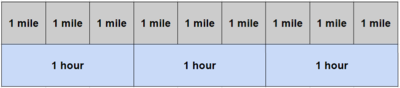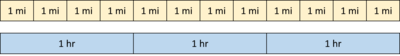# Constant Speed

Alignments to Content Standards: 6.RP.A.3

Callie biked 12 miles in 3 hours. Carter biked 10 miles in 2 hours.

Represent each person's trip with a diagram. Explain how you can see that they are not going the same speed.

## IM Commentary

The purpose of this task is for students to learn to reason about whether or not ratios are equivalent using a diagram. If students are familiar with different types of diagrams, they might be left to decide on an appropriate representation (MP.5). Or a teacher might use this task to practice or promote the use of a particular type of diagram. The type of diagram shown in the solution is a parallel tape diagram, but a double number line diagram or a ratio table could also work nicely if students are familiar with them.

The brief nature of the task statement might suggest this is a nice, short problem for students, but the reasoning could take some time.

If a teacher anticipates that students might struggle to get started, she might preface the task statement with an example using a preferred representation. So before students tackle this task, the class might work to make sense of a statement like, "If you walked 9 miles in 3 hours, we might represent that situation with this diagram."The teacher could ask students what they notice, or ask more specific questions like,

• How much time did it take you to walk 6 miles? [2 hours]
• About how far had you walked after an hour and a half? [$4\frac{1}{2}$ miles]
• If someone were walking slower than you, what would have to be true? [Answers will vary, but involve walking a shorter distance in the same time (8 miles in 3 hours), or taking more time to walk the same distance (9 miles in 4 hours).]

For students who might finish early, some appropriate extension questions might be:

• Suppose someone biked $15\frac{1}{2}$ miles in 4 hours. Is this person going faster or slower than the people in the task? Explain how you know. [This person's speed is $3\frac{7}{8}$ miles per hour, so it's slower than both people in the task.]
• Write a different story about two people biking different distances at the same speed, and draw a diagram that shows how you know they are traveling the same speed.
• Draw a diagram that shows a person biking at a certain speed for one hour, then at a faster speed for the next hour, and then at an even faster speed for the third hour.

The task makes an assumption that each cyclist is traveling at a constant speed. If it comes up, a teacher could just say, "Let's assume that they each travel the same speed for the whole ride."

## Solution

Here is a diagram representing Callie's ride:Here is a diagram representing Carter's ride:Sample reasoning based on comparing the same amount of time: In the first diagram, you can see Callie rides 4 miles in one hour. In the second diagram you can see that Carter rides 5 miles in one hour. Since they each travel a different distance in the same amount of time, they are not traveling at the same speed.

Sample reasoning based on comparing the same distance: In the first diagram, you can see that Callie takes 1 hour to travel 4 miles. But in the second diagram, you can see that Carter takes less than one hour to travel 4 miles. Since it takes them a different amount of time to travel the same distance, they are not traveling at the same speed.